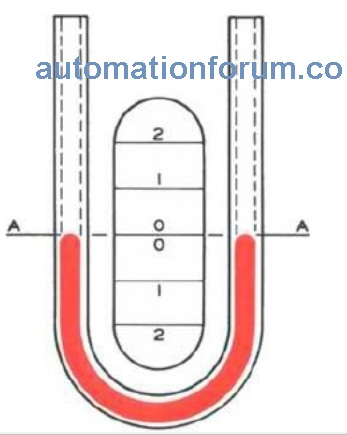# Different types of Manometers## Introduction

There are variety of pressure sensing elements. Manometer is one of the pressure sensors used to sense very low level pressure scales.we are going to discuss about manometers in this session

## The U Manometer

The manometer is a simple device to measure small amounts of pressure.  It consists of a glass tube of a fixed diameter.  It is bent into a U shape with (vertical) sides.  The sides are next to a scale.  The manometer is filled with a liquid, e.g. water or mercury.• Absolute Pressure

One side is sealed with a vacuum above the liquid in the manometer.  An unknown pressure is applied to the other side (limb) which forces the liquid down.  The difference in height (H) of the liquid column will give the unknown absolute pressure.

For example, a 9 inch difference, with water as the liquid, will give an absolute pressure of 9 x 249.1 = 2242 Pa.

• Gauge Pressure

One side is left open to the atmosphere.  An unknown pressure is applied to the other side.  This pressure will force the liquid in the tube down.  The height of the liquid gives the gauge pressure of the unknown pressure.

• Differential Pressure

If unknown pressures are applied to both sides the difference in level (H) will give the difference (differential) between the two in absolute pressure.

## The Well ManometerThe well manometer  is like the U tube manometer.  It is used to measure very low pressures.  The pressure is measured in inches of water (H2O).  This measurement is divided by the ratio of the areas A and B.  This gives the unknown pressure.  A single limb manometer in a workshop usually has its scale already calibrated to allow for the ratio of the areas A and B.  So, no calculations are required.

##The inclined limb manometer  is another device for measuring very low pressures.  The unknown pressure is applied to the well and the single limb is tilted.  This makes the scale longer so the pressure can be measured more accurately.  The actual pressure is the height, H.  No calculations are required as the scale is set by the manufacturer to give accurate readings

## Dual tube ManometerA dual tube manometer is a manometer that is designed to read very high pressures. A high pressure causes the need for a longer indicating tube, which is very inconvenient to the person reading the manometer. A dual-tube manometer solves this problem by having two tubes to read the pressure, a standard well-type manometer and a well-type manometer with the well at the 100-inch reading on the indicating tube.